Examples of solution of stiff differential equations by explicit methods

Homepage

# Dumka3 Fortran examples

• Solution of Brusselator equation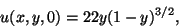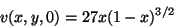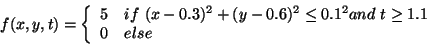sources:

# Dumka3 C examples

• Solution of Nagumo nerve conduction equation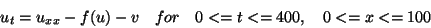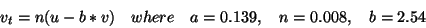with initial condition: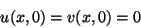and boundary conditions: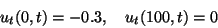The function f is defined by :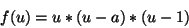sources:
• Convection-diffusion equation with a source term

(u_t + 0.5*sigma^2*x^2*u_xx + (r-D_0)*x*u_x - ru) = 0
and Linear Complementary problem for American Call
(u_t + 0.5*sigma*x^2*u_xx + (r-D_0)*x*u_x - ru)*(u(x,t) - Call(x)) = 0
(u_t + 0.5*sigma^2*x^2*u_xx + (r-D_0)*x*u_x - ru) >= 0
(u(x,t) - C(x)) >= 0
Final condition C(x) = max(x-E,0) (initial condition)
E = 10.e0
D0 = 0.2e0
r = 0.25e0
sigma = 0.8e0
The conservative form of the equation:
u_t + (0.5*sigma^2*x^2*u_x +(r-D_0-sigma^2)*x*u)_x - -(r-D_0-sigma^2)*u - ru = 0 solutions:
• Integro-Differential Convection-Diffusion Equation: dB/dt = mean_reversion_r(r0-r)*dB/dr + mean_reversion_lambda(lambda0-lambda)*dB/dlambda + 0.5*sigma_r^2*d^2B/dr^2 + 0.5*sigma_lambda^2*d^2B/dlambda^2 + rho*sigma_r*sigma_lambda*d^2B/dr*dlambda -(r+lambda)*B + g(t,r,lambda)*lambda + f(t,r,lambda), where B=B(t,x,lambda)=E[exp(-\int^T_0 x(t)+lambda(t) dt ] - x and intensity lambda. We assume process: mean-reverting Ornstein Uhlenbeck model and jump of Poisson process N(t)==1 with intensity lambda, g(t,r,lambda) - expected value of integral term

# Dumka3 C++ examples

• Solution of y'=-a(y-cos(t)):
sources:

solutions:

discussion:

• We compare explicit Euler method and dumka3 by time of computation for the same accuracy of the result. We do not compare implicit method and dumka3 for this simple problem, because it is obvious that implicit method will be faster for this particular example, because it is very fast to inverse matrix in this particular case of one-dimensions problem. The situation is completely different for problems that appear after semidiscretization of large 2-3 dimensional systems of partial differential equations. (See further examples.)
In this example you can see that Euler method with stable(!) step size 2/a is not accurate at all, so it makes sence to compare these two methods for the same tolerance. To achieve the same tolerance approximately 0.1 step size in Euler method was 1/a. Acceleration obtained by dumka3 for a=500 was about 2.3, and for a=5000 it was about 14.5
• Solution of Burgers equation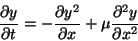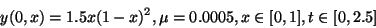:
sources:

solutions:

discussion:

• Solution of Brusselator equationsources:

solutions:

discussion:

•